#### DiracTrick

##### Description

DiracTrick[exp] contracts gamma matrices with each other and performs several simplifications, but no expansion, use Calc or DiracSimplify for non-commutative expansion.

``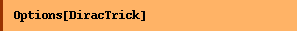``
`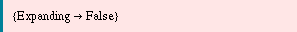`

##### Examples

This is a string of Dirac matrices in four dimensions.

``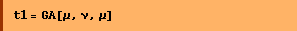``
`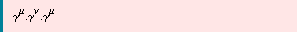`
``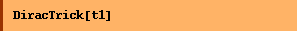``
`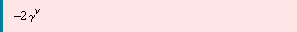`

This is a string of Dirac matrices in D dimensions.

``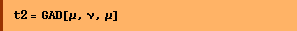``
``
``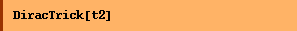``
`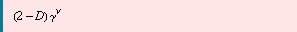`
``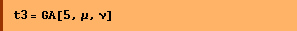``
`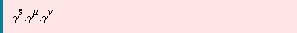`

By default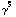is moved to the right.

``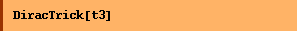``
`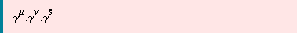`
``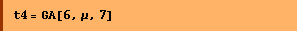``
`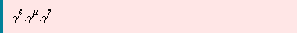`
``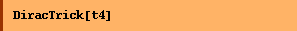``
`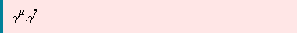`
``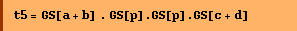``
`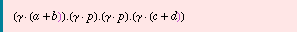`
``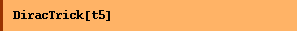``
`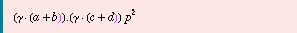`
``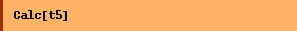``
`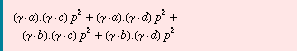`
``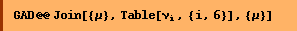``
`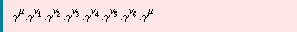`
``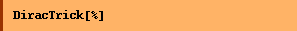``
`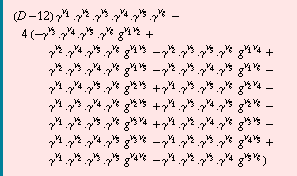`

Converted from the Mathematica notebook DiracTrick.nb